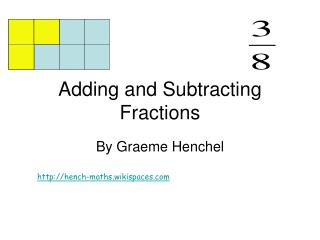DownloadDownload PresentationTélécharger la présentation- - - - - - - - - - - - - - - - - - - - - - - - - - - E N D - - - - - - - - - - - - - - - - - - - - - - - - - - -
##### Presentation Transcript

1. Adding and Subtracting Fractions By Graeme Henchel http://hench-maths.wikispaces.com

2. Adding Fractions with common denominators

3. Adding Fractions with different denominators Problem: You can’t add fractions with different denominators without getting them ready first. They will be ready to add when they have common denominators Solution: Turn fractions into equivalent fractions with a common denominator that is find the Lowest Common Multiple (LCM) of the two denominators

4. Finding the Lowest Common Denominator • The lowest common multiple of two numbers is the lowest number in BOTH lists of multiples Multiples of 2 are 2, 4, 6, 8, 10…… Multiples of 3 are 3, 6, 9, 12, ……… What is the lowest common multiple?

5. Finding the Lowest Common Denominator • The lowest common multiple of two numbers is the lowest number they will BOTH divide into 2 divides into 2, 4, 6, 8….. 3 divides into 3, 6, 9…. What is the lowest number 2 and 3 both divide into?

6. You can’t add fractions with different denominators + The Lowest Common Multiple of 2 and 3 is 6 so turn all fractions into sixths Special form of 1

7. Lowest common denominator is 10 so make all fractions tenths

8. Turn both fractions into twelfths

9. Finally the fractions are READY to add. I just have to add the numerators 9+14=23 It is 3/3 So I multiply 3/7 by 3/3 It is 7/7 So I multiply 2/3 by 7/7 What is the lowest number BOTH 3 and 7 divide into? Hmmmmm?????? What special form of 1 will change 7 to 21. Hmmmm? What special form of 1 will change 3 to 21. Hmmmm? It is 21. So that is my common denominator Now 3x3=9 and 2x7=14 Now I know the new numerators

10. Adding Mixed Numbers • Separate the fraction and the whole number sections, add them separately and recombine at the end